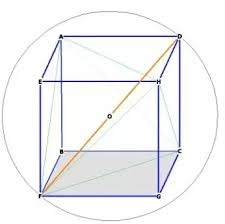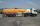# The volume

The volume of the sphere is 1 m3, what is its surface?

Correct result:

S =  4.836 m2

#### Solution:We would be pleased if you find an error in the word problem, spelling mistakes, or inaccuracies and send it to us. Thank you!#### You need to know the following knowledge to solve this word math problem:

We encourage you to watch this tutorial video on this math problem:

## Next similar math problems:

• Sphere A2VThe surface of the sphere is 241 mm2. What is its volume?
• Cone - from volume surface areaThe volume of the rotating cone is 1,018.87 dm3, its height is 120 cm. What is the surface area of the cone?
• Area of a cubeCalculate the surface area of a cube if its volume is equal to 729 cubic meters.
• The regularThe regular quadrilateral pyramid has a volume of 24 dm3 and a height of 45 cm. Calculate its surface.
• EightEight small Christmas balls with a radius of 1 cm have the same volume as one large Christmas ball. What has a bigger surface: eight small balls, or one big ball?
• Chemical parisonThe blown parison (with shape of a sphere) have a volume 1.5 liters. What is its surface?
• Iron sphereIron sphere has weight 100 kg and density ρ = 7600 kg/m3. Calculate the volume, surface and diameter of the sphere.
• The ballThe ball has a radius of 2m. What percentage of the surface and volume is another sphere whose radius is 20% larger?
• Sphere cutA sphere segment is cut off from a sphere k with radius r = 1. The volume of the sphere inscribed in this segment is equal to 1/6 of the volume of the segment. What is the distance of the cutting plane from the center of the sphere?
• Gasoline tank cylindricalWhat is the inner diameter of the tank, which is 8 m long and contains 40 cubic cubic meters of gasoline?
• Surface areaThe volume of a cone is 1000 cm3 and the content area of the axis cut is 100 cm2. Calculate the surface area of the cone.The surface of the sphere is 60 cm square. Calculate its radius; result round to tenth of cm.A sphere with a diameter of 20.6 cm, the cut is a circle with a diameter of 16.2 cm. .What are the volume of the segment and the surface of the segment?The cube has a surface of 384 cm2. Calculate its volume.The surface of the sphere is 12100 cm2, and the weight is 136 kg. What is its density?The volume of a cube is 27 cubic meters. Find the height of the cube.The surface of the cube is 500 cm2, how much cm3 will be its volume?